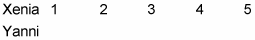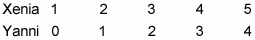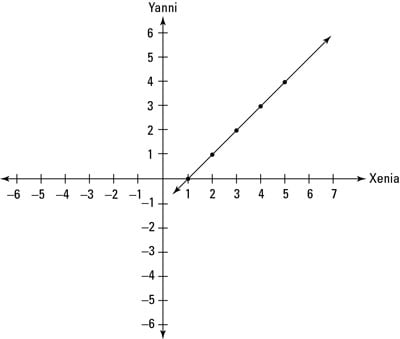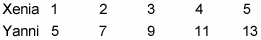##### Trigonometry Workbook For DummiesBy plotting points on a Cartesian graph, you can draw lines between these points and use them to show mathematical relationships. The following examples focus on the number of dollars that two people, Xenia and Yanni, are carrying. The horizontal axis represents Xenia’s money, and the vertical axis represents Yanni’s. For example, suppose you want to draw a line representing this statement:

Xenia has \$1 more than Yanni.

To do this, make a chart as follows:Now fill in each column of the chart assuming that Xenia has that number of dollars. For example, if Xenia has \$1, then Yanni has \$0. And if Xenia has \$2, then Yanni has \$1. Continue until your chart looks like this:Now you have five pairs of points that you can plot on your graph as (Xenia, Yanni): (1, 0), (2, 1), (3, 2), (4, 3), and (5, 4). Next, draw a straight line through these points, as shown in the below figure.All possible values of Xenia’s and Yanni’s money if Xenia has \$1 more than Yanni.
This line on the graph represents every possible pair of amounts for Xenia and Yanni. For example, notice how the point (6, 5) is on the line. This point represents the possibility that Xenia has \$6 and Yanni has \$5.

Here’s a slightly more complicated example:

Yanni has \$3 more than twice the amount that Xenia has.

Again, start by making the usual chart:You can fill in this chart by supposing that Xenia has a certain amount of money and then figuring out how much money Yanni would have in that case. For example, if Xenia has \$1, then twice that amount is \$2, so Yanni has \$3 more than that, or \$5. And if Xenia has \$2, then twice that amount is \$4, so Yanni has \$3 more, or \$7. Continue in that way to fill in the chart as follows:Now you can plot these five points on the graph and draw a line through them.